Although the CMBR is close to being an isotropic and thermal radiation background with simple spectral and angular distributions, it is useful to recall the formalism needed to deal with a general radiation field, since the details of the small perturbations have great physical significance. The notation used here is similar to that of Shu (1991), which may be consulted for more detailed descriptions of the quantities employed.

The state of a radiation field can be described by distribution functions f(r,p,t), such that the number of photons in real space volume d3r about r and momentum space volume d3p about p at time t with polarization(= 1 or 2) is fd3r d3p. This distribution function is related to the photon occupation number in polarization state, n(r,p,t), by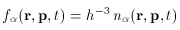(13)

and to the specific intensity in the radiation, I(k, ^r, t), by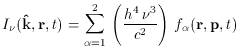(14)

where k ^ is a unit vector in the direction of the radiation wavevector,is the photon frequency, and h and c are Planck's constant and the speed of light. The meaning of the specific intensity is that the energy crossing area element dS in time dt from within solid angle dabout k ^ and with frequency in the rangeto+ dis I(k ^ · dS) dddt.

If the occupation number is of Planck form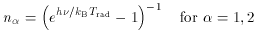(15)

then the radiation field has the form of (1). The number density of photons in the Universe is then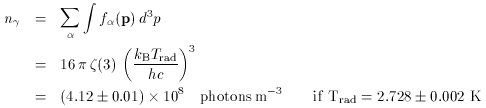(16)

from which can be calculated the baryon to photon number ratio,= nB/n= 2.7 x 10-8B h1002. In (16)(x) is the Riemann zeta function ((3)1.202) and the value of Trad is taken from a recent analysis of COBE data on the CMBR spectrum (Fixsen et al. 1996).

Similarly, the energy density of the radiation field is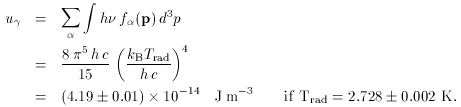(17)

It is apparent that the errors on uand nin (16) and (17) are so small as to have no significant astrophysical impact, and may safely be dropped.

It is common for the specific intensity of a radiation field to be described by radio-astronomers in units of brightness temperature, TRJ. This is defined as the temperature of a thermal radiation field which in the Rayleigh-Jeans limit of low frequency would have the same brightness as the radiation that is being described. In the limit of low frequency (1) reduces to I= 2 KB Trad2 / c2, so that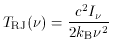(18)

Thus the brightness temperature of a thermal spectrum as described by (1) is frequency-dependent, with a peak value equal to the radiation temperature at low frequencies, and tending to zero in the Wien tail.

In the presence of absorption, emission and scattering processes, and in a flat spacetime, Iobeys a transport equation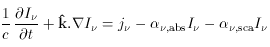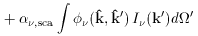(19)

where jis the emissivity along the path (the energy emitted per unit time per unit frequency per unit volume per unit solid angle),,abs is the absorption coefficient (the fractional loss of intensity of the radiation per unit length of propagation because of absorption by material in the beam),,sca is the scattering coefficient (the fractional loss of intensity of the radiation per unit length of propagation because of scattering by material in the beam), and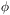(^k, k' ^) is the scattering redistribution function - the probability of a scattering from direction ^ k' to ^ k. The absorption coefficient is regarded as containing both true absorption and simulated emission. While this is important in astrophysical masers, where,abs is negative, this subtlety will not affect the discussions in the present review. An important property of Ithat follows from its definition (or equation 19) is that it is conserved in flat spacetimes in the absence of radiation sources or absorbers.

The specific intensity of a radiation field may be changed in several ways. One is to make the photon distribution function anisotropic, for example by the Doppler effect due to the peculiar motion of the Earth relative to the sphere of last scattering, which causes the radiation temperature becomes angle-dependent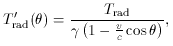(20)

but otherwise leaves the form of (15) unchanged.= [1 - (v2 / c2)]-1/2 and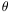is the angle between the line of sight and the observer's velocity vector (Peebles & Wilkinson 1968). The specific intensity may also be changed by redistributing photons to different directions and frequencies (e.g., by scattering processes), or by absorbing or emitting radiation (e.g., by thermal bremsstrahlung). The choice of whether to describe these effects in the photon distribution function, or in the specific intensity, is made for reasons of convenience. Although the statistical mechanics of photon scattering is often related to the occupation numbers, n, most astrophysical work is done in the context of the specific intensity, I.